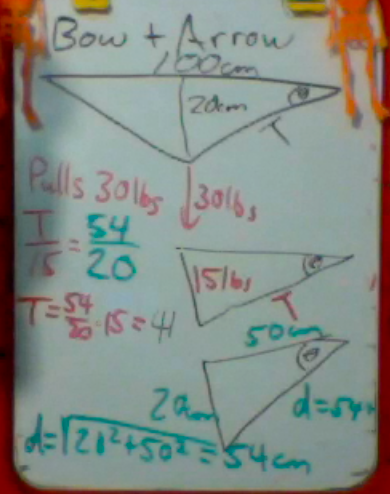# Strings and Things

We are going to look at Statics, the study of stationary objects in two dimensions. Many of these examples involve strings.

In our first example, consider a picture frame with a mass of 5 kg.  The force of gravity is then 50 N.

There are two strings, each with a tension T.The weight of the picture frame is supported equally by both strings, so each string has to only support 25 N of weight.

The tension can be broken into vertical and horizontal components. The vertical component of the tension is equal and opposite of half of the weight.  The horizontal components of the tension work against each other.  Since the angle is 45°, then those components are equal.  Using the Pythagorean theorem, we find that the tension in each string is 33N.Anyone notice how the suspended tape measure from the Marsh Chapel experiment was hanging in the wind?Suppose we hung a 2 kg weight from the tape measure, which is 20 meters long.  The tape measure then sags a vertical distance of 30 cm.  What is the tension in the tape measure?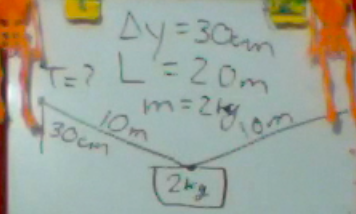We can set up two similar vector diagrams (like similar triangles).  One diagram for force, the other for displacement.

The force diagram has Tension as the hypotenuse, and supports half of the weight, or half of 20 Newtons.

The displacement vector diagram has an opposite side of 30 cm = 0.3 m and a hypotenuse of 10 m.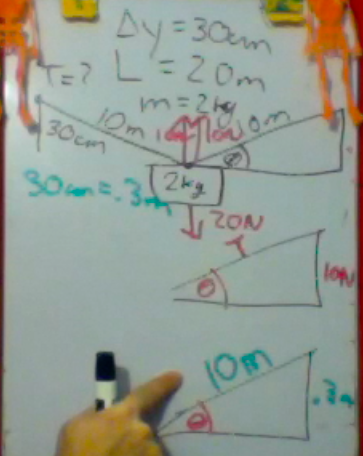We do NOT need trig to solve this problem.  We can solve it using similar triangles. Using the fact that the lengths of the sides are proportional, we set up a simple ratio.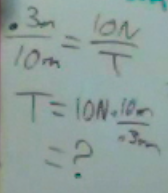For our last example.For his Birthday this past August, I treated Alejandro to an archery lesson.

Suppose we model the bow as a triangle.  Okay, Alejandro is shooting a compound bow, but it is a model.

Suppose the bow is 100 cm long, and Alejandro pulls with 30 pounds of force. If he can pull the bow back 20 cm, what is the tension in the bow string?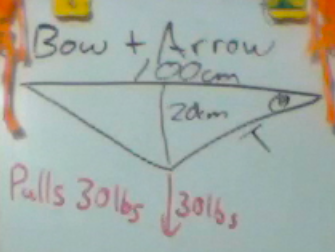We set up similar vector diagram as in the previous problem.  One for force, and the other for displacement. Note, his applied force is split between the two sides of the string.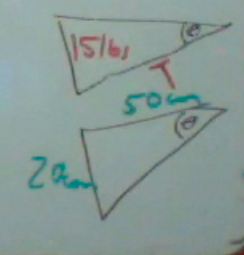Now when you go to solve this problem, there is an extra step.  You do not have the necessary sides of the triangle.  So you also need to use the Pythagorean theorem to find the length of the string.  Given the length of the string is 54 cm, then you can set up your ratios to find the tension in the bow, which is about 41 pounds.